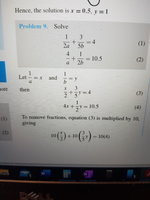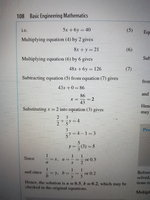# Simultaneous equations (or shall I say system of equations)

#### Yuseph

##### Junior Member
Hey guys wussup,

I had to stop there because I accumulated two sticking points.
1) the author never explained why he sometimes used a substraction and why sometimes an addition. Is it simply a matter of whether one of the letter is gonna be removed at the end. Or is there a case where you use substraction and another only addition ?
2) why does the guy bother with his letter replacements ? I dont care about that i want to cross multiply quickly and finish with the stuff. Is he right or not ?
3) why am I not getting the same result as him ? It is the author who lost himself with his replacement stuff or am I doing sth wrong ?#### Attachments

• 2.7 MB Views: 2

#### Subhotosh Khan

##### Super Moderator
Staff member
Hey guys wussup,

I had to stop there because I accumulated two sticking points.
1) the author never explained why he sometimes used a substraction and why sometimes an addition. Is it simply a matter of whether one of the letter is gonna be removed at the end. Or is there a case where you use substraction and another only addition ?
2) why does the guy bother with his letter replacements ? I dont care about that i want to cross multiply quickly and finish with the stuff. Is he right or not ?
3) why am I not getting the same result as him ? It is the author who lost himself with his replacement stuff or am I doing sth wrong ?
View attachment 21611View attachment 21612
View attachment 21614
Where did the green-highlighted stuff come from?

#### Yuseph

##### Junior Member
Where did the green-highlighted stuff come from?
?
Its number 2
There are two equations in the question

#### HallsofIvy

##### Elite Member
I am puzzled by this. You say "the author"- what author? What textbook? Also what part of what you post here is from the text book and what part is your work? For the first problem, on the left, we have "43b= 59" which gives $$\displaystyle b= \frac{59}{43}$$ (which cannot be reduced) but on the right, the "check", $$\displaystyle b= \frac{1}{5}$$!

At the very first you have the equation $$\displaystyle \frac{1}{2a}+ \frac{3}{5b}= 4$$ and immediately below it 5a+ 6b= 4. Where did that second equation come from? Yes, to "eliminate the fractions" you want to multiply by the least common denominator, and here that is (2a)(5b)= 10ab. Multiplying the left side by 10ab will eliminate the denominators on the left- $$\displaystyle \frac{1}{2a}(10ab)+ \frac{3}{5b}(10ab)= 5b+ 6a$$ but you have to multiply the right side of the equation by that same thing. You should have $$\displaystyle 5b+ 6a= 4(10ab)= 40ab$$.

Similarly, you have $$\displaystyle \frac{4}{a}+ \frac{1}{2b}= 10.5$$. You multiply by the "common denominator", 2ab and the left side becomes 8b+ a as you have. but the right side is still "10.5"! You have to multiply the right side of the equation by 2ab also to get 8b+ a= 21ab.

Now you have 5b+ 6a= 40ab and 8b+ a= 21ab. That "ab" on the right of the equations complicates eliminating one or the other of the variables. I can multiply the second equation by 6 to get 48b+ 6a= 126ab (again, you have to do the same thing to both sides of the equation). Now subtract 5b+ 6a= 40ab from that to get 43b= 86ab. We still have both a and b in the equation but, as long as b is not 0, we can divide both sides by b to get 43= 86a and then divide both sides by 86 to get $$\displaystyle a= \frac{1}{2}$$. Now 5b+ 6a= 5b+ 3= 20b so 15b= 3, $$\displaystyle b= \frac{3}{15}= \frac{1}{5}$$.

As far as "letter replacements" are concerned that is just another method to simplify the problem. You say "I dont care about that i want to cross multiply quickly and finish with the stuff. Is he right or not ?" The problem is you didn't "cross multiply" and apparently don't know what that really means! You cant "cross multiply" on just one side of the equations. When you say "is he right or not" if you mean making those substitutions is necessary, no they are not- I did the problem without those substitutions. But some people don't like that or just do it wrong- like you did. By letting x= 1/a and y=1/b we get linear equations, x/2+ 3y/5= 4 and 4x+ y/2= 10.5. Most people learn equations like that first and are comfortable with them before dealing with problems where the variables are not in the denominators of fractions.[/tex]

•Yuseph

#### Yuseph

##### Junior Member
- The book is Basic engineering mathematics 6th edition
- Thats nothing i was just trying to compare his method of replacement with cross multiplying but since you said it cant be done on just one side and i didnt have that information thats ok now
- Is there any way i can cross multiply 'first' instead of eliminating the denominators ?

#### Attachments

• 5.7 MB Views: 4

#### Yuseph

##### Junior Member
And what about the substraction between the two equations ? Because in some exercices they add to each other and some exercice the author substract ? Does it follow a rule or is it not important as long as one of the unknown can be removed ?

#### Subhotosh Khan

##### Super Moderator
Staff member
And what about the substraction between the two equations ? Because in some exercices they add to each other and some exercice the author substract ? Does it follow a rule or is it not important as long as one of the unknown can be removed ?
"........it is not important as long as one of the unknown can be removed" - Correct

•Yuseph

#### JeffM

##### Elite Member
Cross multiplication is a short cut for clearing fractions when there are just two fractions involved, one on each side of the equal sign

$$\displaystyle \dfrac{2}{p} = \dfrac{5}{q} \implies \cancel pq * \dfrac{2}{\cancel p} = p \cancel q * \dfrac{5}{\cancel q} \implies 2q = 5p.$$

It only works in that very special case. The method of clearing fractions that works in all cases is multiplication of both sides of the equation by the product of the denominators (or the least common multiple of all the denominators).

The general method to solve systems of simultaneous equations is by substitution. A solution by elimination is NEVER needed. It's useful if you quickly see a way to do it, in which case it makes no difference whether you use addition or subtraction.

•Yuseph